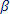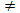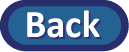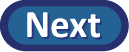## Theory-Based Approach

Because the null distribution of sample slopes is approximately normal, we can standardize the sample slope into a t-statistic and use the t-distribution to estimate the p-value. (There are of course technical conditions to check for this, but we aren't going to worry about them too much this time.)

In the applet, above the null distribution use the Statistic pull-down menu to select the t-statistic optio . Check the new box to Overlay t-distribution.

(o) Enter the test statistic you calculated in (m) and press Count. (Don't worry if the applet complains about your value, could be rounding differences.) Does the theoretical p-value provide a reasonable approximation to the simulated p-value?

Now check the box under the original scatterplot to display the Regression Table. Click here for an explanation of the regression output. Take a screen capture of this output (null distribution and regression table) and copy it into your report.

(p) How does the SE value corresponding to the friends row compare to the standard deviation of the slopes found in (j)? (Note: They may not be all that close because they are actually based on slightly different assumptions, but they do both aim to estimate the same thing.)

(q) How does this t-value compare to what you found in (m)?

(r) How does the p-value in the output for the Regression Table compare to the one-sided p-value you estimated in (k)?

Note: The t value in the friends row is our test statistic and the p-value reported there is actually always the two-sided p-value for testing H0:= 0 vs. Ha:0. When you have a one-sided alternative hypothesis, you can take the p-value reported by in the regression output and cut it in half to determine the one-sided p-value.

(s) Check the box underneath the Regression Table to display the theory-based 95% Confidence interval for slope. How does it compare to the interval you found in (n)? Write a one-sentence interpretation of this interval.